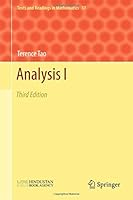# Analysis I: 3rd Edition1 Reviews
2016-07-20
350 pages

## Book Description

This is part one of a two-volume book on real and is intended for senior undergraduate students of mathematics who have already been exposed to . The emphasis is on rigour and foundations of . Beginning with the construction of the number systems and set , the book discusses the basics of analysis (limits, series, continuity, differentiation, Riemann ), through to power series, several variable calculus and , and then finally the Lebesgue integral. These are almost entirely set in the concrete setting of the real line and Euclidean spaces, although there is some material on abstract metric and topological spaces. The book also has appendices on mathematical and the decimal system. The entire text (omitting some less central topics) can be taught in two quarters of 25-30 lectures each. The course material is deeply intertwined with the exercises, as it is intended that the student actively learn the material (and practice thinking and writing rigorously) by proving several of the key results in the .

Chapter 1 Introduction
Chapter 2 Starting at the beginning: the natural numbers
Chapter 3 Set theory
Chapter 4 Integers and rationals
Chapter 5 The real numbers
Chapter 6 Limits of sequences
Chapter 7 Series
Chapter 8 Infinite sets
Chapter 9 Continuous functions on R
Chapter 10 Differentiation of functions
Chapter 11 The Riemann integral
Appendix A: the basics of mathematical logic
Appendix B: the decimal system

## Book Details

• Title: Analysis I: 3rd Edition
• Author:
• Length: 350 pages
• Edition: 3
• Language: English
• Publisher:
• Publication Date: 2016-07-20
• ISBN-10: 9811017883
• ISBN-13: 9789811017889